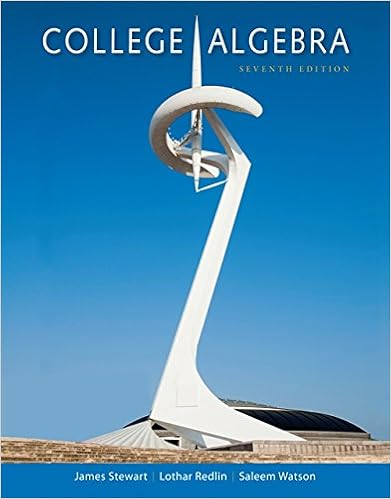# 3 at a construction site a pipe wrench struck the

• 30

This preview shows page 23 - 30 out of 30 pages.

##### We have textbook solutions for you!
The document you are viewing contains questions related to this textbook.The document you are viewing contains questions related to this textbook.
Chapter P / Exercise 5
College Algebra
Redlin/StewartExpert Verified
3. At a construction site a pipe wrench struck the ground with a speed of 24 m/s. a) From what height was it inadvertently dropped? b). How long was it falling? Problems
4. A key falls from a bridge that is 45 m above the water. It falls directly into a model boat, moving with constant velocity that is 12 m from the point of impact when the key is released. What is the speed of the boat?
##### We have textbook solutions for you!
The document you are viewing contains questions related to this textbook.The document you are viewing contains questions related to this textbook.
Chapter P / Exercise 5
College Algebra
Redlin/StewartExpert Verified
1. How far does an automobile move while its speed increases uniformly from 15 kph to 45 kph in 20 seconds? 2. Cars A and B are traveling in the same direction at the same speed of 70 km/hr in a level road. Car A is 150 m ahead of car B. Car A decelerates at a constant rate of 2 m/s 2 . From this instant up to the time B overtakes A , how far has B traveled ? 3. An car starting from rest speeds up to 40 ft/s with a constant acceleration of 4 ft/s 2, runs at this speed for a time and finally comes to rest with a deceleration of 5 ft/s 2 . If the total distance traveled is 1000 ft, find the total time required. Problems (Seatwork)
4. A stone is thrown vertically upward an returns to earth in 10 seconds. How high did it go and what is its initial velocity? 5. A ball is thrown vertically upward with an initial velocity of 3m/s from a window of a tall building. The ball strikes the ground 4 seconds later. Determine the height of the window above the ground. Problems (Seatwork)
Acceleration as Function of Time and Velocity Sample Problem 1 A Ford Mustang moves in a straight line such that for a short time its velocity is defined by v = (3t 2 + 2t) ft/s, where t is in seconds. Determine its position and acceleration when t = 3 s. When t = 0, s = 0 .
Acceleration as Function of Time and Velocity Sample Problem 2 A particle moving along a straight line is subjected to a deceleration a = ( -2v 3 ) m/s 2 , where v is in m/s. If it has a velocity v = 8 m/s and a position s = 10 m when t = 0, determine its velocity and position when t = 4 s.
Acceleration as Function of Time Homework
Acceleration as Function of Time
Seatwork 2. A particle travels along a straight line with a velocity v = (12 3t 2 ) m/s, where t is in seconds. When (t = 1 s, the particle is located 10 m to the left of the origin. Determine the acceleration when t = 4 s, the displacement from t = 0 to t = 10 s, and the distance the particle travels during this time period.
•••